## grid recipes

these snippets of code are starting points for some of the most common grid interactions, to help make incorporating grids into your scripts easier.

each are written following these “house” techniques:

• use flags to determine when to redraw the grid
• use tables to track flag states and grid coordinates
• query flag states at 30fps and only redraw when things change

these microstudies have been designed with simplicity and extensibility in mind. drop them into an existing script and stitch an interface together, or start from an interface and fill in functions as you go!

(grid GIFs created using `@Tyler`’s excellent GridCapture library)

## TOC

### simple redraw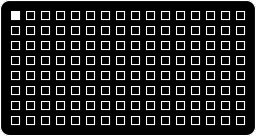all keys are set up as switches, so only one can be lit at a time. press a new one, it lights up as the previous selection goes out. useful for state changes. use encoder 3 to change brightness level.

core concepts:

• `clock`-centric grid redraw
• `grid_dirty` flag to prompt grid redraw
• introduce table elements (`show.x` and `show.y` instead of variables)
``````g = grid.connect() -- 'g' represents a connected grid

function init()
brightness = 15 -- brightness = full bright!
show = {x = 1, y = 1} -- table tracking x,y position
grid_dirty = true -- initialize with a redraw
clock.run(grid_redraw_clock) -- start the grid redraw clock
end

function grid_redraw_clock() -- our grid redraw clock
while true do -- while it's running...
clock.sleep(1/30) -- refresh at 30fps.
if grid_dirty then -- if a redraw is needed...
grid_redraw() -- redraw...
grid_dirty = false -- then redraw is no longer needed.
end
end
end

function grid_redraw() -- how we redraw
g:all(0) -- turn off all the LEDs
g:led(show.x,show.y,brightness) -- light this coordinate at indicated brightness
g:refresh() -- refresh the hardware to display the new LED selection
end

function g.key(x,y,z) -- define what happens if a grid key is pressed or released
if z==1 then -- if a grid key is pressed down...
show.x = x -- update stored x position to selected x position
show.y = y -- update stored y position to selected y position
grid_dirty = true -- flag for a redraw
end
end

function enc(n,d) -- define what happens in an encoder is turned
if n==3 then -- if encoder 3 is turned...
brightness = util.clamp(brightness + d,0,15) -- inc/dec brightness
grid_dirty = true -- flag for a redraw
end
end
``````

try this:

• start the script with a random x,y position
• control brightness with a different encoder
• when a grid key is released, execute `print("key released!")`

### momentary keys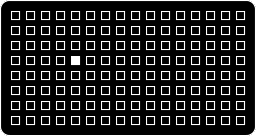classic earthsea-style interaction. press a key and it lights up as it’s held. release to extinguish.

the difference between this snippet and the ‘simple redraw’ is that the state of every key is being independently tracked. this means that the state of each key won’t influence the others – instead of only one lit key at a time, you can press many keys at once and they’ll all light up.

core concepts:

• establish a table that holds booleans for every grid key
• utilize inline conditions (see line 41)
``````g = grid.connect() -- 'g' represents a connected grid

function init()
grid_dirty = false -- script initializes with no LEDs drawn

momentary = {} -- meta-table to track the state of all the grid keys
for x = 1,16 do -- for each x-column (16 on a 128-sized grid)...
momentary[x] = {} -- create a table that holds...
for y = 1,8 do -- each y-row (8 on a 128-sized grid)!
momentary[x][y] = false -- the state of each key is 'off'
end
end

clock.run(grid_redraw_clock) -- start the grid redraw clock
end

function grid_redraw_clock() -- our grid redraw clock
while true do -- while it's running...
clock.sleep(1/30) -- refresh at 30fps.
if grid_dirty then -- if a redraw is needed...
grid_redraw() -- redraw...
grid_dirty = false -- then redraw is no longer needed.
end
end
end

function grid_redraw() -- how we redraw
g:all(0) -- turn off all the LEDs
for x = 1,16 do -- for each column...
for y = 1,8 do -- and each row...
if momentary[x][y] then -- if the key is held...
g:led(x,y,15) -- turn on that LED!
end
end
end
g:refresh() -- refresh the hardware to display the LED state
end

function g.key(x,y,z)  -- define what happens if a grid key is pressed or released
-- this is cool:
momentary[x][y] = z == 1 and true or false -- if a grid key is pressed, flip it's table entry to 'on'
-- what ^that^ did was use an inline condition to assign our momentary state.
-- same thing as: if z == 1 then momentary[x][y] = true else momentary[x][y] = false end
grid_dirty = true -- flag for redraw
end
``````

try this:

• restrict momentary keys to a 64-sized grid
• restrict momentary keys to even-numbered rows

### toggles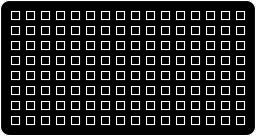press an unlit key and it toggles on at half-bright. hold a half-bright key to make it full-bright. hold it again to return to half-bright. press a half-bright key to toggle it off.

here, we enter state management territory. instead of defining single-state toggles for each key, we use additional gesture information to switch between two toggle states: half-bright and full-bright. this is the foundation of modified behavior.

core concepts:

• using `clock` to track held time
• managing `clock` state to cancel clocks with unmet criteria
• modifying existing states
``````g = grid.connect()

function init()
grid_dirty = false

toggled = {} -- meta-table to track the state of the grid keys
brightness = {} -- meta-table to track the brightness of each grid key
counter = {} -- meta-table to hold counters to distinguish between long and short press
for x = 1,16 do -- for each x-column (16 on a 128-sized grid)...
toggled[x] = {} -- create an x state tracker,
brightness[x] = {} -- create an x brightness,
counter[x] = {} -- create a x state counter.
for y = 1,8 do -- for each y-row (8 on a 128-sized grid)...
toggled[x][y] = false -- create a y state tracker,
brightness[x][y] = 15 -- create a y brightness.
-- counters don't need futher initialization because they start as nil...
-- counter[x][y] = nil
end
end

clock.run(grid_redraw_clock)
end

function g.key(x,y,z)
if z == 1 then -- if a grid key is pressed...
counter[x][y] = clock.run(long_press,x,y) -- start the long press counter for that coordinate!
elseif z == 0 then -- otherwise, if a grid key is released...
if counter[x][y] then -- and the long press is still waiting...
clock.cancel(counter[x][y]) -- then cancel the long press clock,
short_press(x,y) -- and execute a short press instead.
end
end
end

function short_press(x,y) -- define a short press
if not toggled[x][y] then -- if the coordinate isn't toggled...
toggled[x][y] = true -- toggle it on,
brightness[x][y] = 8 -- set brightness to half.
elseif toggled[x][y] and brightness[x][y] == 8 then -- if the coordinate is toggled and half-bright
toggled[x][y] = false -- toggle it off.
-- we don't need to set the brightness to 0, because off LED will not be turned back on once we redraw
end
grid_dirty = true -- flag for redraw
end

function long_press(x,y) -- define a long press
clock.sleep(0.5) -- a long press waits for a half-second...
-- then all this stuff happens:
if toggled[x][y] then -- if key is toggled, then...
brightness[x][y] = brightness[x][y] == 15 and 8 or 15 -- flip brightness 8->15 or 15->8.
end
counter[x][y] = nil -- clear the counter
grid_dirty = true -- flag for redraw
end

function grid_redraw()
g:all(0)
for x = 1,16 do
for y = 1,8 do
if toggled[x][y] then -- if coordinate is toggled on...
g:led(x,y,brightness[x][y]) -- set LED to coordinate at specified brightness.
end
end
end
g:refresh()
end

function grid_redraw_clock()
while true do
if grid_dirty then
grid_redraw()
grid_dirty = false
end
clock.sleep(1/30)
end
end
``````

try this:

• modify the script so that a long press on an unlit key toggles the key at full-bright (current behavior: only a short press can toggle an unlit key)
• modify the script so that a short press on full-bright key drops to half-bright (current behavior: only a long press on a full-bright key will drop to half-bright)

### state machine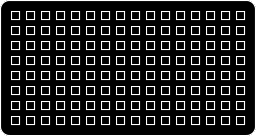sorta like toggles, but a long press momentarily inverts the state of the key whereas a short press flips the state. very useful for alt menus + modifiers and doesn’t require dedicating any real-estate to single-purpose “meta” keys.

core concepts:

• implementing a low-level state machine
• using `clock` to track held time
• managing `clock` state to cancel clocks with unmet criteria
``````g = grid.connect()

function init()
grid_dirty = true

toggled = {} -- meta-table to track the toggled state of each grid key
alt = {} -- meta-table to track the alt state of each grid key
counter = {}

for x = 1,16 do -- 16 cols
toggled[x] = {}
alt[x] = {}
counter[x] = {}
for y = 1,8 do -- 8 rows
toggled[x][y] = false
alt[x][y] = false
-- counters don't need futher initialization because they start as nil
end
end

clock.run(grid_redraw_clock)
end

function g.key(x,y,z)
if z == 1 then -- if a key is pressed...
counter[x][y] = clock.run(long_press,x,y) -- start counting toward a long press.
elseif z == 0 then -- if a key is released...
if counter[x][y] then -- if the long press counter is still active...
clock.cancel(counter[x][y]) -- kill the long press counter,
short_press(x,y) -- because it's a short press.
else -- if there was a long press...
long_release(x,y) -- release the long press.
end
end
end

function long_press(x,y)
clock.sleep(0.25) -- 0.25 second press = long press
alt[x][y] = true
counter[x][y] = nil -- set this to nil so key-up doesn't trigger a short press
grid_dirty = true
end

function long_release(x,y)
alt[x][y] = false
grid_dirty = true
end

function short_press(x,y)
toggled[x][y] = not toggled[x][y]
grid_dirty = true
end

function grid_redraw()
g:all(0)
for x=1,16 do
for y=1,8 do
if toggled[x][y] and not alt[x][y] then
g:led(x,y,15)
elseif alt[x][y] then
g:led(x,y,toggled[x][y] == true and 0 or 15)
end
end
end
g:refresh()
end

function grid_redraw_clock()
while true do
if grid_dirty then
grid_redraw()
grid_dirty = false
end
clock.sleep(1/30)
end
end
``````

try this:

• short press = half-bright, long press = full-bright
• only during a long press, draw additional LEDs
• allow new toggles to be entered during a long press which are only redrawn during long presses

### switches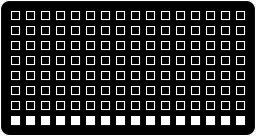foundation for a step sequencer – 16 columns of switches, stealing vertically.

core concepts:

• using nested tables to segment the grid display
``````g = grid.connect()

function init()
grid_dirty = true
switch = {}
for i = 1,16 do -- since we want rows to steal from each other, we only set up unique indices for columns
switch[i] = {y = 8} -- equivalent to switch[i]["y"] = 8
end
clock.run(grid_redraw_clock)
end

function grid_redraw_clock()
while true do
if grid_dirty then
grid_redraw()
grid_dirty = false
end
clock.sleep(1/30)
end
end

function grid_redraw()
g:all(0)
for i = 1,16 do
g:led(i, switch[i].y, 15)
end
g:refresh()
end

function g.key(x,y,z)
if z == 1 then
switch[x].y = y
grid_dirty = true
end
end
``````

try this:

• incorporate a long press to modify and display an additional table of switches

### range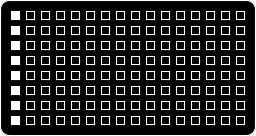hold a grid key and press another in the same row to establish a range. pressing a single key will establish a new start point for the range, so long as the entire range can fit. establishing a negative range resets to 1.

core concepts:

• using nested tables with multiple elements to track state
• using if/then conditions to define all possible interactions
``````g = grid.connect()

function init()
grid_dirty = true
range = {}
for i = 1,8 do
range[i] = {x1 = 1, x2 = 1, held = 0} -- equivalent to range[i]["x1"], range[i]["x2"], range[i]["held"]
end
clock.run(grid_redraw_clock)
end

function grid_redraw_clock()
while true do
clock.sleep(1/30)
if grid_dirty then
grid_redraw()
grid_dirty = false
end
end
end

function grid_redraw()
g:all(0)
for y = 1,8 do
for x = range[y].x1, range[y].x2 do
g:led(x,y,15)
end
end
g:refresh()
end

function g.key(x,y,z)
if z == 1 then
range[y].held = range[y].held + 1 -- tracks how many keys are down
local difference = range[y].x2 - range[y].x1
local original = {x1 = range[y].x1, x2 = range[y].x2} -- keep track of the original positions, in case we need to restore them
if range[y].held == 1 then -- if there's one key down...
range[y].x1 = x
range[y].x2 = x
if difference > 0 then -- and if there's a range...
if x + difference <= 16 then -- and if the new start point can accommodate the range...
range[y].x2 = x + difference -- set the range's start point to the selectedc key.
else -- otherwise, if there isn't enough room to move the range...
-- restore the original positions.
range[y].x1 = original.x1
range[y].x2 = original.x2
end
end
elseif range[y].held == 2 then -- if there's two keys down...
range[y].x2 = x -- set an range endpoint.
end
if range[y].x2 < range[y].x1 then -- if our second press is before our first...
range[y].x2 = range[y].x1 -- destroy the range.
end
elseif z == 0 then -- if a key is released...
range[y].held = range[y].held - 1 -- reduce the held count by 1.
end
grid_dirty = true
end
``````

try this:

• incorporate a long press to reset the range to 1

### meters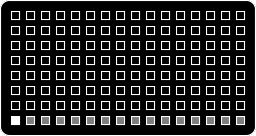16 vertical meters, use E3 to change height. great for step sequencers or showing parameter + variable states.

core concepts:

• more advanced table nesting + methods
• reverse `for` count
• `g:led` features in-line comparison
``````g = grid.connect()

function init()
grid_dirty = true
meter = { {} , selected = 1 }
for x = 1,16 do
meter[x] = {height = 0}
end
clock.run(grid_redraw_clock)
end

function grid_redraw_clock()
while true do
clock.sleep(1/30)
if grid_dirty then
grid_redraw()
grid_dirty = false
end
end
end

function grid_redraw()
g:all(0)
for x = 1,16 do
for y = 8,8-meter[x].height,-1 do
g:led(x,y,meter.selected == x and 15 or 7)
end
g:led(meter.selected,8,15)
end
g:refresh()
end

function g.key(x,y,z)
if z == 1 then
meter.selected = x
end
grid_dirty = true
end

function enc(n,d)
if n == 3 then
meter[meter.selected].height = util.clamp(meter[meter.selected].height+d,0,7)
grid_dirty = true
end
end
``````

try this:

• add a moving playhead indicator across the grid which runs across the top-most key in each column
• add a mechanism to the bottom row which dynamically sets a range to restrict the playhead’s movement
• add long presses as a way to toggle between two different meter states